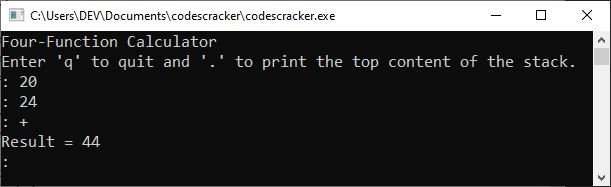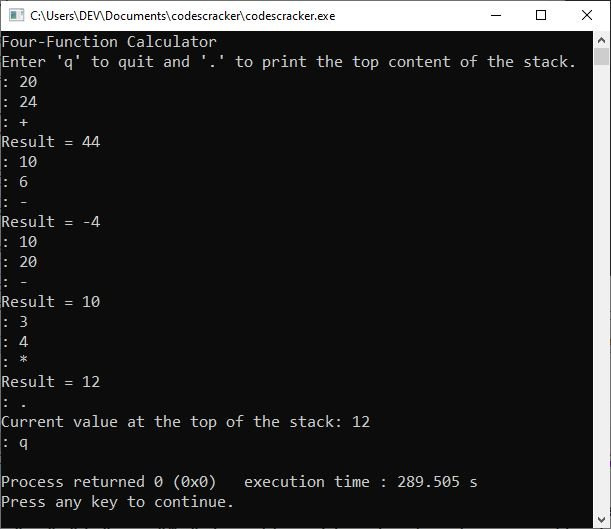# Stack data structure in C programming with an example program

Because I defined the "stack" data structure in a separate post, this post is solely dedicated to implementing the stack data structure in C. So, without further ado, let us begin with a brief overview.

## A brief introduction to the stack data structure

A stack is different from a queue in that it works on the "last-in, first-out" (LIFO) principle, where a queue works on "first-in, first-out" (FIFO). Therefore, we can say that the "stack" is just the opposite of a queue.

To visualize a stack, just imagine a stack of plates. The first plate on the table is the last to be used, and the last plate placed on the stack is the first to be used. Stacks are used frequently in system software, including compilers and interpreters.

## Stack implementation in the C programming language

When working with stacks, the two basic operations, i.e., store and review, are traditionally called push and pop, respectively. Therefore, to implement a stack, you need two functions (one responsible for storing and the other one responsible for reviewing), i.e., the push() function (which places a value on the stack) and the pop() function (which retrieves a value from the stack). You also need a region of memory to use as a stack. You can use an array for this purpose or allocate a region of memory using the dynamic allocation functions of C. As with the queue, the retrieval function takes a value off the list and destroys it if it is not stored elsewhere. The general form of push() and pop() that use an integer array follows. You can maintain the stacks of other data types by changing the base type of the array on which the push() and pop() functions operate.

## The general forms of push() and pop()

Here is the general form of the functions push() and pop().

```int stack[MAX];
int top = 0;

void push(int i)
{
if (top >= MAX)
{
printf("Stack Overflow!\n");
return;
}
stack[top] = i;
top++;
}
int pop(void)
{
top--;
if (top < 0)
{
printf("Stack Underflow!\n");
return 0;
}
return stack[top];
}```

The variable "top" is the index of the stack's top. When implementing these functions, keep the stack from overflowing and underflowing in mind. An empty stack is indicated by "top" being zero, and a full stack is indicated by "top" being greater than the last storage location.

## Stack's operation

Here is a table that shows how a stack works:

Action Stack Contents
push(A) A
push(B) B A
push(C) C B A
pop() retrieves C B A
push(F) F B A
pop() retrieves F B A
pop() retrieves B A
pop() retrieves A empty

The above table shows the stack in action. That is how a stack works in C.

## C Stack Example

Now is the time to create an example before closing the discussion on the implementation of the stack data structure in the C programming language to make the post fully understandable. This is a four-function calculator program that shows how the stack works.

```#include <stdio.h>

#define MAX 100

int *p;              // point to a region of free memory
int *top;            // points to the top of the stack
int *bot;            // points to the bottom of the stack

void push(int);
int pop(void);

int main()
{
int x, y;
char str;

p = (int *)malloc(MAX * sizeof(int));    // obtain stack memory
if (!p)
{
printf("Allocation Failure!\n");
return 0;
}
top = p;
bot = p + MAX - 1;

printf("Four-Function Calculator\n");
printf("Enter 'q' to quit and '.' to print the top content of the stack.\n");

do
{
printf(": ");
gets(str);
switch (*str)
{
case '+':
x = pop();
y = pop();
printf("Result = %d\n", x + y);
push(x + y);
break;
case '-':
x = pop();
y = pop();
printf("Result = %d\n", x - y);
push(x - y);
break;
case '*':
x = pop();
y = pop();
printf("Result = %d\n", x * y);
push(x * y);
break;
case '/':
x = pop();
y = pop();
if (x == 0)
{
printf("Divide-by-zero error!\n");
break;
}
printf("Result = %d\n", y / x);
push(y / x);
break;
case '.':            // Show the content of the stack's top in this case.
x = pop();
push(x);
printf("Current value at the top of the stack: %d\n", x);
break;
default:
push(atoi(str));
}
} while (*str != 'q');

return 0;
}

// put an element on the stack
void push(int i)
{
if (p > bot)
{
printf("Stack Overflow..!!\n");
return;
}
*p = i;
p++;
}

// retrieve the top element from the stack
int pop(void)
{
p--;
if (p < top)
{
printf("Stack Underflow!\n");
return 0;
}
return *p;
}```

The following snapshot shows the initial output produced by the above program, demonstrating the "stack implementation in C."Now, type a number, such as 20, and press the ENTER key. Then, type another number, such as 24, and press the ENTER key again. Finally, type any operator, such as "+," "-," "/," or "*," to add, subtract, divide, or multiply. For example, after entering the two numbers, I typed "+" as the operator and pressed the ENTER key; the result is shown below.You can either continue the calculation operation or type "." and press the ENTER key to see the content of the top of the stack and "q" to exit the program. The sample run is depicted in the following snapshot:C Online Test

« Previous Tutorial Next Tutorial »